#Riccati EquationsBefore we give the formal definition of Riccati equations, a little introduction may be helpful. Indeed, consider the first order differential equation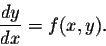If we approximate f(x,y), while x is kept constant, we will get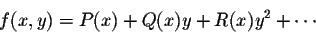If we stop at y, we will get a linear equation. Riccati looked at the approximation to the second degree: he considered equations of the type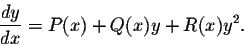These equations bear his name, Riccati equations. They are nonlinear and do not fall under the category of any of the classical equations. In order to solve a Riccati equation, one will need a particular solution. Without knowing at least one solution, there is absolutely no chance to find any solutions to such an equation. Indeed, let y1 be a particular solution ofConsider the new function z defined by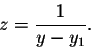Then easy calculations give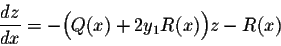which is a linear equation satisfied by the new function z. Once it is solved, we go back to y via the relation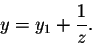Keep in mind that it may be harder to remember the above equation satisfied by z. Instead, try to do the calculations whenever you can.

Example. Solve the equation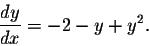knowing that y1 = 2 is a particular solution.

Answer. We recognize a Riccati equation. First of all we need to make sure that y1 is indeed a solution. Otherwise, our calculations will be fruitless. In this particular case, it is quite easy to check that y1 = 2 is a solution. Set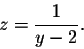Then we have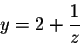which implies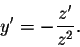Hence, from the equation satisfied by y, we get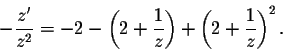Easy algebraic manipulations give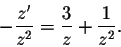Hence

z' = -3z -1.

This is a linear equation. The general solution is given by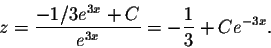Therefore, we have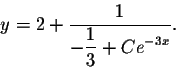Note: If one remembers the equation satisfied by z, then the solutions may be found a bit faster. Indeed in this example, we have P(x) = -2, Q(x) = -1, and R(x) = 1. Hence the linear equation satisfied by the new function z, is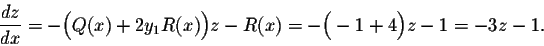Example. Check that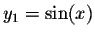is a solution to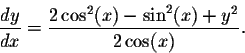Then solve the IVP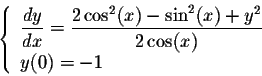We will let the reader check that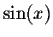is indeed a particular solution of the given differential equations. We also recognize that the equation is of Riccati type. Set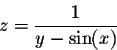which gives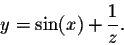Hence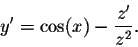Substituting into the equation gives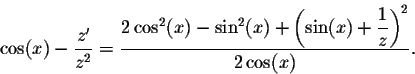Easy algebraic manipulations give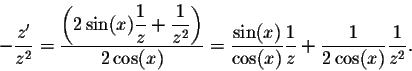Hence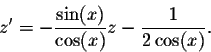This is the linear equation satisfied by z. The integrating factor is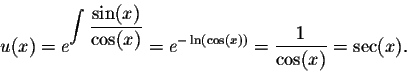The general solution is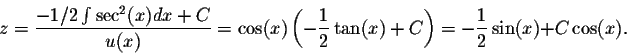Now it is time to go back to the original function y. We have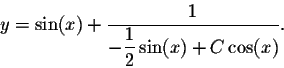The initial condition y(0) = -1 implies 1/C = -1, or C = -1. Therefore the solution to the IVP is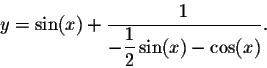[Differential Equations] [Algebra] [Trigonometry ]
[Calculus] [Complex Variables] [Matrix Algebra]S.O.S. MATHematics home page

Do you need more help? Please post your question on our S.O.S. Mathematics CyberBoard.Author: Mohamed Amine Khamsi
Last Update 9-15-98